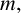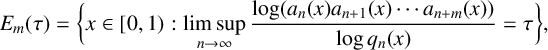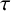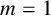Hostname: page-component-7d8f8d645b-2q4x6 Total loading time: 0 Render date: 2023-05-29T20:21:41.354Z Has data issue: false Feature Flags: { "useRatesEcommerce": true } hasContentIssue false

## Abstract

This paper is concerned with the growth rate of the product of consecutive partial quotients relative to the denominator of the convergent for the continued fraction expansion of an irrational number. More precisely, given a natural number$m,$ we determine the Hausdorff dimension of the following set:\begin{align*} E_m(\tau)=\bigg\{x\in [0,1): \limsup\limits_{n\rightarrow\infty}\frac{\log (a_n(x)a_{n+1}(x)\cdots a_{n+m}(x))}{\log q_n(x)}=\tau\bigg\}, \end{align*}

where$\tau$ is a nonnegative number. This extends the dimensional result of Dirichlet nonimprovable sets (when$m=1$ ) shown by Hussain, Kleinbock, Wadleigh and Wang.

## MSC classification

Type
Research Article
Information
Journal of the Australian Mathematical Society , December 2022 , pp. 357 - 385
© The Author(s), 2021. Published by Cambridge University Press on behalf of Australian Mathematical Publishing Association Inc.

## Access options

Get access to the full version of this content by using one of the access options below. (Log in options will check for institutional or personal access. Content may require purchase if you do not have access.)

## Footnotes

This work was supported by NSFC (Grant Nos. 12001190 and 11871208) and the Science and Technology Development Fund, Macau SAR (no. 0024/2018/A1).

## References

Bakhtawar, A., ‘Hausdorff dimension for the set of points connected with the generalized Jarník–Besicovitch set’, J. Aust. Math. Soc., to appear. Published online (7 December 2020).Google Scholar
Bakhtawar, A., Bos, P. and Hussain, M., ‘The sets of Dirichlet non-improvable numbers versus well-approximable numbers’, Ergodic Theory Dynam. Systems 40(12) (2020), 32173235.CrossRefGoogle Scholar
Bakhtawar, A., Bos, P. and Hussain, M., ‘Hausdorff dimension of an exceptional set in the theory of continued fractions’, Nonlinearity 33(6) (2020), 26152639.Google Scholar
Besicovitch, A. S., ‘Sets of fractional dimension (IV): on rational approximation to real numbers’, J. Lond. Math. Soc. 9(2) (1934), 126131.CrossRefGoogle Scholar
Bos, P., Hussain, M. and Simmons, D., ‘The generalised Hausdorff measure of sets of Dirichlet non-improvable numbers’, Preprint, 2020, arXiv:2010.14760.Google Scholar
Bugeaud, Y., ‘Sets of exact approximation order by rational numbers’, Math. Ann. 327(1) (2003), 171190.CrossRefGoogle Scholar
Davenport, H. and Schmidt, W. M., ‘ Dirichlet’s theorem on Diophantine approximation ,’ in: Symposia Mathematica (INDAM, Rome, 1968/69), Vol. IV (Academic Press, London, 1970), 113132.Google Scholar
Falconer, K. J., Fractal Geometry: Mathematical Foundations and Applications, 2nd edn (John Wiley & Sons, Hoboken, NJ, 2003).CrossRefGoogle Scholar
Good, I. J., ‘The fractional dimensional theory of continued fractions’, Proc. Cambridge Philos. Soc. 37 (1941), 199228.CrossRefGoogle Scholar
Haas, A., ‘The relative growth rate for partial quotients’, New York J. Math. 14 (2008), 139143.Google Scholar
Huang, L. and Wu, J., ‘Uniformly non-improvable Dirichlet set via continued fractions’, Proc. Amer. Math. Soc. 147(11) (2019), 46174624.CrossRefGoogle Scholar
Huang, L., Wu, J. and Xu, J., ‘Metric properties of the product of consecutive partial quotients in continued fractions’, Israel J. Math. 238(2) (2020), 901943.CrossRefGoogle Scholar
Hussain, M., Kleinbock, D., Wadleigh, N. and Wang, B.-W., ‘Hausdorff measure of sets of Dirichlet non-improvable numbers’, Mathematika 64(2) (2018), 502518.Google Scholar
Iosifescu, M. and Kraaikamp, C., Metrical Theory of Continued Fractions, Mathematics and Its Applications, 547 (Kluwer Academic Publishers, Dordrecht, 2002).CrossRefGoogle Scholar
Jarník, I., ‘Zur metrischen theorie der diophantischen approximationen’, Prace Mat. Fiz. 36 (1928), 91106.Google Scholar
Jarník, V., ‘Diophantische Approximationen und Hausdorffsches Mass’, Mat. Sb. 36 (1929), 371382.Google Scholar
Khintchine, A. Ya., Continued Fractions (Noordhoff, Groningen, 1963), translated by P. Wynn.Google Scholar
Khintchine, A. Ya., Continued Fractions (University of Chicago Press, Chicago, IL, 1964).Google Scholar
Kleinbock, D. and Wadleigh, N., ‘A zero-one law for improvements to Dirichlet’s theorem’, Proc. Amer. Math. Soc. 146(5) (2018), 18331844.CrossRefGoogle Scholar
Mauldin, D. and Urbański, M., ‘Dimensions and measures in infinite iterated function systems’, Proc. Lond. Math. Soc. (3) 73(1) (1996), 105154.CrossRefGoogle Scholar
Sun, Y. and Wu, J., ‘A dimensional result in continued fractions’, Int. J. Number Theory 10(4) (2014), 849857.CrossRefGoogle Scholar
Tan, B. and Zhou, Q., ‘The relative growth rate for partial quotients in continued fractions’, J. Math. Anal. Appl. 478(1) (2019), 229235.CrossRefGoogle Scholar
Wang, B.-W. and Wu, J., ‘Hausdorff dimension of certain sets arising in continued fraction expansions’, Adv. Math. 218(5) (2008), 13191339.CrossRefGoogle Scholar
Wang, B.-W., Wu, J. and Xu, J., ‘A generalization of the Jarník–Besicovitch theorem by continued fractions’, Ergodic Theory Dynam. Systems 36(4) (2016), 12781306.CrossRefGoogle Scholar